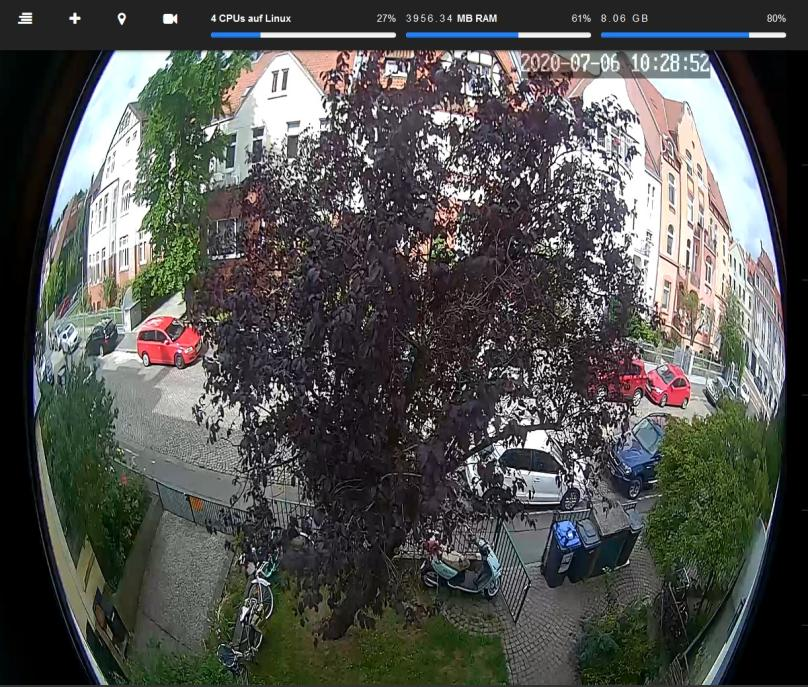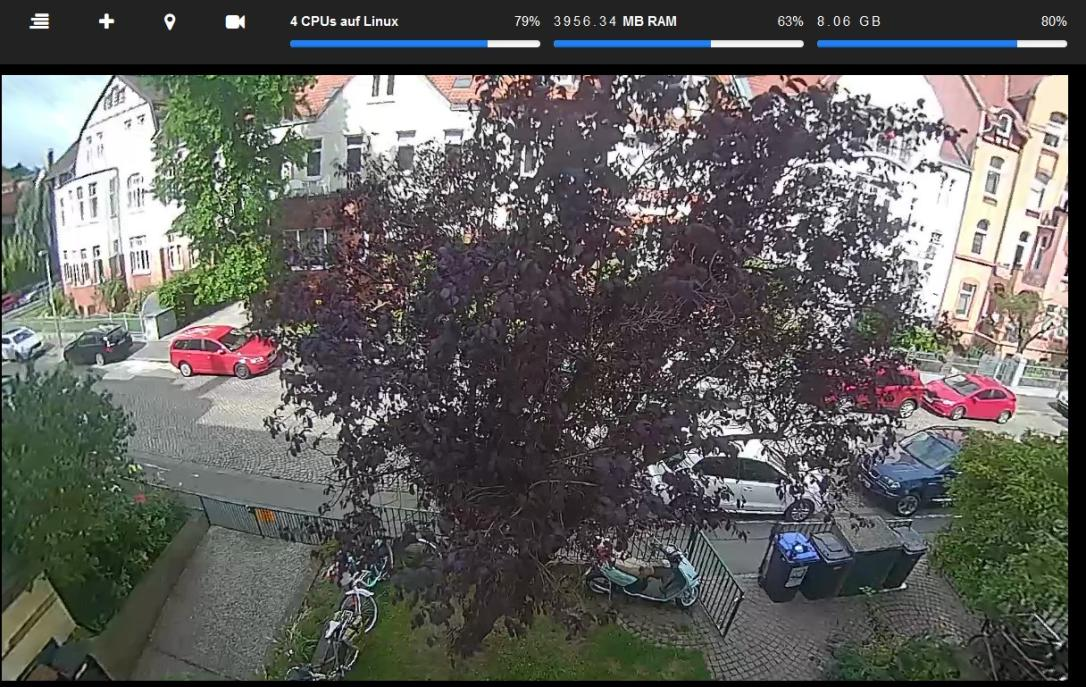## Going to the Stream Settings and select MJPEG as Type1. Go to the Camera Settings
2. Click on Stream
3. Select MJPEG as Stream Type
4. Copy and paste this Settings into the Video Filter
`"lenscorrection=cx=0.5:cy=0.5:k1=-0.227:k2=-0.022"`

Copy the complete String, including the "" (quotes).

Note, that this will increase the CPU Usage from 20% to around 80% on a Jetson Nano.

cx

Relative x-coordinate of the focal point of the image, and thereby the center of the distortion. This value has a range [0,1] and is expressed as fractions of the image width. Default is 0.5.

cy

Relative y-coordinate of the focal point of the image, and thereby the center of the distortion. This value has a range [0,1] and is expressed as fractions of the image height. Default is 0.5.

k1

Coefficient of the quadratic correction term. This value has a range [-1,1]. 0 means no correction. Default is 0.

k2

Coefficient of the double quadratic correction term. This value has a range [-1,1]. 0 means no correction. Default is 0.

The formula that generates the correction is:

`r_src = r_tgt * (1 + k1 * (r_tgt / r_0)^2 + k2 * (r_tgt / r_0)^4)`

where r_0 is halve of the image diagonal and r_src and r_tgt are the distances from the focal point in the source and target images, respectively.